# Difference between revisions of "Limiting coefficient of static friction"

## Definition

Given two surfaces, the limiting coefficient of static friction (also called the coefficient of static friction or static friction coefficient) between them, denoted$\mu_s$ or$\mu_l$, is defined as the number such that the static friction between any two bodies with these two surfaces respectively as the surfaces of contact cannot exceed$\mu_sN$, where$N$ is the normal force between the two bodies.

For a block resting on a table, the limiting coefficient of static friction between the lower surface of the block and the upper surface of the table is defined as the maximum possible ratio of an applied horizontal force to normal force for which the block does not slip against the table.

The notion of limiting coefficient of static friction arises as part of the Coulomb model of friction.

The angle$\theta$ defined as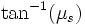$\tan^{-1}(\mu_s)$ is termed the angle of friction.

## Units and dimensions

The limiting coefficient of static friction is a dimensionless scalar and does not need units.

## Constitutive factors and measurement

### Constitutive factors

The limiting coefficient of static friction between two surfaces depends on the two surfaces, and as such, should be the same for the same pair of surfaces. However, the coefficient depends on a number of factors such as dampness, adherence of dust to the surfaces, scratches, or minor surface changes, hence the measurement for the same pair of surfaces may differ with time.

### Directional dependence

The limiting coefficient of static friction may vary somewhat with direction if the surfaces themselves are not isotropic, i.e., they appear different in different directions. The microscopic corrugations of surfaces such as wood may be different in different directions.

### Empirical measurement

For this reason, the limiting coefficient of static friction for a pair of surfaces must be determined empirically, through measurement. However, a reasonable approximate value can be estimated based on the nature of the surfaces.

## Coefficient of static friction for composite of surfaces

Because the surfaces may be non-uniform, the limiting coefficient of static friction may be different for different parts of the surface. In this case, there is no single choice of an average limiting coefficient of static friction. Rather, the limiting value of static friction that can be applied also depends on how the normal force is distributed across the different parts of the surface. If$P$ denotes the pressure, then the limiting value of static friction is the integral over area elements:$\int \mu_s(A) P \, dA$

## Range of values

The limiting coefficient of static friction typically takes values in the range of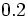$0.2$ to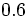$0.6$. In most cases, the limiting coefficient of static friction is less than$1$, but there could be situations where it is greater than$1$. For very slippery surfaces, the limiting coefficient of static friction may be less than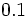$0.1$.

First surface Second surface Condition$\mu_s$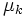$\mu_k$$\tan^{-1}(\mu_s)$ (radians)$\tan^{-1}( \mu_s)$ (degrees)$\tan^{-1}(\mu_k)$ (radians)$\tan^{-1}(\mu_k)$ (degrees)
Aluminimum Mild steel Dry and clean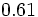$0.61$$0.47$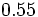$0.55$$31$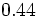$0.44$$25$
Aluminimum Aluminimum Dry and clean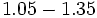$1.05 - 1.35$$1.4$$0.81 - 0.93$$46 - 53$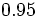$0.95$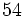$54$
Copper Steel Dry and clean$0.53$$0.36$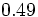$0.49$$28$$0.35$$20$
Copper Copper Dry and clean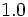$1.0$  ?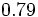$0.79$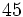$45$  ?  ?
Wood Concrete Dry and clean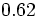$0.62$  ?$0.55$$32$  ?  ?
Wood Metal Dry and clean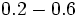$0.2 - 0.6$  ?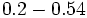$0.2 - 0.54$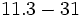$11.3 - 31$  ?  ?
Wood Metal Wet$0.2$  ?$0.2$$11.3$
Wood Wood Dry and clean$0.25 - 0.5$  ?$0.24 - 0.46$$14 - 26$  ?  ?
Wood Wood Wet$0.2$  ?$0.2$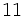$11$  ?  ?
Mild steel Mild steel Dry and clean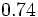$0.74$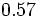$0.57$$0.64$$37$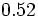$0.52$$30$
Hard steel Hard steel Dry and clean$0.78$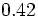$0.42$$0.66$$38$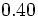$0.40$$23$

For more, see The Engineer's Handbook and Engineering Toolbox.

## Subtleties

### Coefficient of static friction makes sense only for uniform surface of contact

The coefficient of static friction makes sense only when the nature of the contact surfaces is uniform throughout the area of contact. If the surface is nonuniform, different parts of the surface may yield different contributions. In this case, the maximum possible value of static friction depends not only on the total value of the normal force but also on the way the normal force is distributed over the surface of contact. If$P$ is the pressure, then: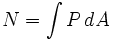$N = \int P \, dA$

and the maximum permissible static friction force is:$f_s = \int \mu_s P \, dA$